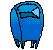Math Fundamentals Geometry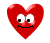1. Find both the the perimeter and area of the following figure: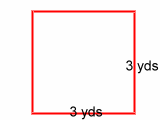2. Find the perimeterof the following figure: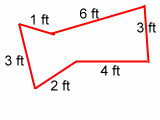3. Find the perimeter of the following figure: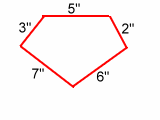4. Find both the perimeter and area of the rectangle:5. Find the perimeter of the following triangle.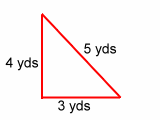6. Find the perimeter and area of the following rectangle: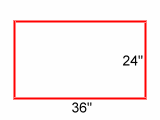7. Find both the perimeter and area of the following figure: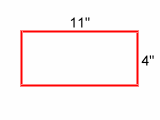8. Find both the perimeter and area of the square: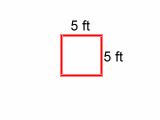9. Find the perimeter of the following figure:10. Find the perimeter of the triangle: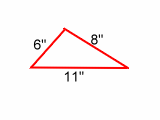11. Find both the perimeter and area of the square:Happy Valentine's Day !!

email